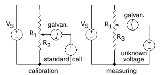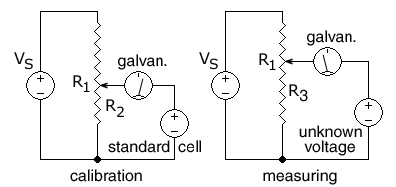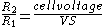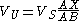xPotentiometer (measuring instrument)Encyclopedia
A potentiometer is an instrument for measuring the potential
Potential
*In linguistics, the potential mood*The mathematical study of potentials is known as potential theory; it is the study of harmonic functions on manifolds...

(voltage) in a circuit. Before the introduction of the moving coil and digital volt meters, potentiometer
Potentiometer
A potentiometer , informally, a pot, is a three-terminal resistor with a sliding contact that forms an adjustable voltage divider. If only two terminals are used , it acts as a variable resistor or rheostat. Potentiometers are commonly used to control electrical devices such as volume controls on...

s were used in measuring voltage
Voltage
Voltage, otherwise known as electrical potential difference or electric tension is the difference in electric potential between two points — or the difference in electric potential energy per unit charge between two points...

, hence the '-meter' part of their name. The method was described by Johann Christian Poggendorff
Johann Christian Poggendorff
Johann Christian Poggendorff , was a German physicist born in Hamburg.By far the greater and more important part of his work related to electricity and magnetism. Poggendorff is known for his electrostatic motor which is analogous to Wilhelm Holtz's electrostatic machine...

around 1841 and became a standard laboratory measuring technique.

In this arrangement, a fraction of a known voltage from a resistive slide wire is compared with an unknown voltage by means of a galvanometer
Galvanometer
A galvanometer is a type of ammeter: an instrument for detecting and measuring electric current. It is an analog electromechanical transducer that produces a rotary deflection of some type of pointer in response to electric current flowing through its coil in a magnetic field. .Galvanometers were...

. The sliding contact or wiper of the potentiometer is adjusted and the galvanometer briefly connected between the sliding contact and the unknown voltage. The deflection of the galvanometer is observed and the sliding tap adjusted until the galvanometer no longer deflects from zero. At that point the galvanometer draws no current from the unknown source, and the magnitude of voltage can be calculated from the position of the sliding contact.

This null balance measuring method is still important in electrical metrology
Metrology
Metrology is the science of measurement. Metrology includes all theoretical and practical aspects of measurement. The word comes from Greek μέτρον , "measure" + "λόγος" , amongst others meaning "speech, oration, discourse, quote, study, calculation, reason"...

and standards work and is also used in other areas of electronics.

Measurement potentiometers are divided into four main classes listed below.

## Constant current potentiometerIn this circuit, the ends of a uniform resistance
Electrical resistance
The electrical resistance of an electrical element is the opposition to the passage of an electric current through that element; the inverse quantity is electrical conductance, the ease at which an electric current passes. Electrical resistance shares some conceptual parallels with the mechanical...

wire R1 are connected to a regulated DC
Direct current
Direct current is the unidirectional flow of electric charge. Direct current is produced by such sources as batteries, thermocouples, solar cells, and commutator-type electric machines of the dynamo type. Direct current may flow in a conductor such as a wire, but can also flow through...

supply VS for use as a voltage divider. The potentiometer is first calibrated
Calibration
Calibration is a comparison between measurements – one of known magnitude or correctness made or set with one device and another measurement made in as similar a way as possible with a second device....

by positioning the wiper (arrow) at the spot on the R1 wire that corresponds to the voltage of a standard cell so thatA standard electrochemical cell
Electrochemical cell
An electrochemical cell is a device capable of either deriving electrical energy from chemical reactions, or facilitating chemical reactions through the introduction of electrical energy. A common example of an electrochemical cell is a standard 1.5-volt "battery"...

is used whose emf is known (e.g. 1.0183 volts for a Weston standard cell).

The supply voltage VS is then adjusted until the galvanometer
Galvanometer
A galvanometer is a type of ammeter: an instrument for detecting and measuring electric current. It is an analog electromechanical transducer that produces a rotary deflection of some type of pointer in response to electric current flowing through its coil in a magnetic field. .Galvanometers were...

shows zero, indicating the voltage on R2 is equal to the standard cell voltage.

An unknown DC voltage, in series with the galvanometer, is then connected to the sliding wiper, across a variable-length section R3 of the resistance wire. The wiper is moved until no current flows into or out of the source of unknown voltage, as indicated by the galvanometer in series with the unknown voltage. The voltage across the selected R3 section of wire is then equal to the unknown voltage. All that remains is to calculate the unknown voltage from the fraction of the length of the resistance wire that was connected to the unknown voltage.

The galvanometer does not need to be calibrated, as its only function is to read zero or not zero. When measuring an unknown voltage and the galvanometer reads zero, no current is drawn from the unknown voltage and so the reading is independent of the source's internal resistance, as if by a voltmeter
Voltmeter
A voltmeter is an instrument used for measuring electrical potential difference between two points in an electric circuit. Analog voltmeters move a pointer across a scale in proportion to the voltage of the circuit; digital voltmeters give a numerical display of voltage by use of an analog to...

of infinite
Infinity
Infinity is a concept in many fields, most predominantly mathematics and physics, that refers to a quantity without bound or end. People have developed various ideas throughout history about the nature of infinity...

resistance.

Because the resistance wire can be made very uniform in cross-section and resistivity, and the position of the wiper can be measured easily, this method can be used to measure unknown DC voltages greater than or less than a calibration voltage produced by a standard cell without drawing any current
Electric current
Electric current is a flow of electric charge through a medium.This charge is typically carried by moving electrons in a conductor such as wire...

from the standard cell.

If the potentiometer is attached to a constant voltage DC supply such as a lead–acid battery, then a second variable resistor (not shown) can be used to calibrate the potentiometer by varying the current through the R1 resistance wire.

If the length of the R1 resistance wire is AB, where A is the (-) end and B is the (+) end, and the movable wiper is at point X at a distance AX on the R3 portion of the resistance wire when the galvanometer gives a zero reading for an unknown voltage, the distance AX is measured or read from a preprinted scale next to the resistance wire. The unknown voltage can then be calculated:## Constant resistance potentiometer

The constant resistance potentiometer is a variation of the basic idea in which a variable current is fed through a fixed resistor. These are used primarily for measurements in the millivolt and microvolt range.

## Microvolt potentiometer

This is a form of the constant resistance potentiometer described above but designed to minimize the effects of contact resistance and thermal emf. This equipment is satisfactorily used down to readings of 1000 nV or so.

## Thermocouple potentiometer

Another development of the standard types was the 'thermocouple potentiometer' especially adapted for temperature measurement with thermocouple
Thermocouple
A thermocouple is a device consisting of two different conductors that produce a voltage proportional to a temperature difference between either end of the pair of conductors. Thermocouples are a widely used type of temperature sensor for measurement and control and can also be used to convert a...

s.

Potentiometers for use with thermocouples also measure the temperature at which the thermocouple wires are connected, so that cold-junction compensation may be applied to correct the apparent measured EMF to the standard cold-junction temperature of 0 degrees C.

## Analytical chemistry

To make a potentiometric determination of an analyte in a solution, the potential of the cell is measured. This measurement must be corrected for the reference and junction potentials. The concentration of the analyte can then be calculated from the Nernst Equation
Nernst equation
In electrochemistry, the Nernst equation is an equation that can be used to determine the equilibrium reduction potential of a half-cell in an electrochemical cell. It can also be used to determine the total voltage for a full electrochemical cell...

. Many varieties of this basic principle exist for quantitative measurements.